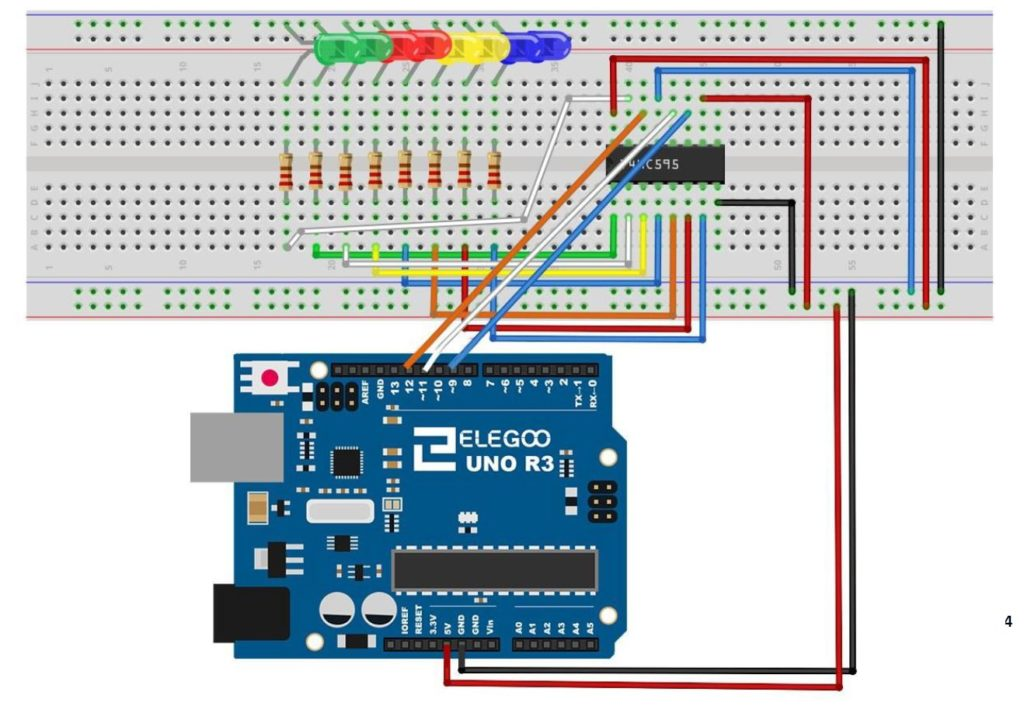# Arduino Tutorial 55: Measuring Distance With HC-SR04 Ultrasonic Sensor

This lesson builds on the work done in Lessons 53 and 55. In this lesson we use the Ultrasonic Sensor to build a distance sensor. The HC-SR04 ultrasonic sensor can be programmed to send out a ping, and then measure the time it takes to hear the echo of the ping come back, after bouncing off a target. Knowing the speed of sound allows us to calculate distance to the sensor.

The circuit uses the following Schematic:

This is the code we develop in the video

The sensor is part of our Elegoo Kit , so if you get this kit, you will be using the same hardware we are using. This project builds on the work we did in Lesson 53.

For this build we will be using an Arduino Nano, which allows the project to be built on a single breadboard. You can use the Arduino Uno if you do not have a Nano, and things will work out the same. The build neatness is also facilitated by using small straight jumper wires, which you can get HERE.

# Arduino Tutorial 51: DHT11 Temperature and Humidity Sensor with LCD Display

In this lesson we work towards a stand alone Temperature and Humidity project using the DHT11 sensor.

This video will take you through the build and coding step-by-step, and the schematics below will help you follow along at home.

Our goal in this lesson is to add an LCD to the project. In lesson 50, we got the sensor working, and here we want to get the LCD connected. We are working with components from the Elegoo kit, which you can get HERE. In addition, your build can be much neater, cleaner and more stable is you use an arduino nano, instead of the standard arduino uno. The nano is small, and has male pins that allow it to be plugged directly into the breadboard, as seen above. I strongly suggest picking up an Arduino Nano, which you can get HERE. This lesson follows on to the work done in lesson 50. To recap, the DHT11 is connected as follows:

You will also need to add the LCD, using the following schematic:

In my photograph at the top of the post, you can see it is important to keep the build neat, as there are lots of connections which must be made. Neat work is facilitated is you use short jumper wires, instead of the big male to male wires. You can get a jumper wire set that will keep your work neat HERE.  I am not trying to sell you a bunch of junk, but as projects get more complicated, you really need to use the short straight wires, or your build will become a rat’s nest.

We develop the code in the video in detail, but you can get the finished code down below.

# Arduino Tutorial 47: Binary and Hexadecimal Bit Flipper

In this lesson we explore how to create a Binary or Hexadecimal Bit Flipper. From our earlier lessons you see we can visually represent Hexadecimal or Binary numbers with a series of LED, with an on LED representing a “1” and an off LED representing a “0”. In programming and circuit applications, it is sometimes useful to “flip” or invert the bits. For an 8 bit number, one could do this in a program with 255 IF statements, but there is a simpler way. If you think about it, you can get the flippedByte by simple subtracting the byte from 0xFF, or 0b11111111. If you try some test cases, you can see that this will always work.

Simply stated, flippedByte=0xFF-Byte,

or if you prefer thinking in binary,

flippedByte=0b11111111-Byte

This is the circuit we are using to drive the 8 LED with the 74HX595 chip, and all this was explained in Tutorial 42.This is the schematic we use in this example to control 8 LEDs from the 74HC595 chip.

This is the code which we developed in the video above.

In all these lessons we are using the Arduino Super Starter Kit, which you can pick up HERE.

# Arduino Tutorial 40: Controlling DC Motor Speed and Direction with Pushbuttons

In this lesson we explore how to control the speed and direction of a DC motor using two buttons. We are using the L293D motor controller and a small DC motor for demonstration purposes. We are using parts from our Elegoo Super Stater Kit, which you can get HERE. The basic circuit was explained in Lesson 37, and we are using that work as a starting point. The schematic below will get you started in connecting your circuit. Be sure and connect one of the Arduino ground pins to the ground rail in the second to the bottom row in the diagram below. It is good practice to connect all your grounds together.

The code we developed in the video lesson is shown below for your convenience.

# Arduino Tutorial 35: Understanding How to Use a Stepper Motor

In this lesson we give you several examples of how to connect and program a stepper motor. Stepper motors are useful because they can produce very large torque at low RPM and are capable of extremely precise positioning. They are somewhat tricky to use, and you must be careful to not try and power them from an Arduino. Arduino can control stepper motors, but they must be powered from an external power supply.

If you want to follow along at home, you can order the Arduino Kit we are using HERE.

This is the code that allows you to toggle the direction of the stepper motor by pressing a pushbutton. The video shows all the details and how to connect the motor up.